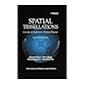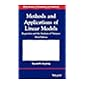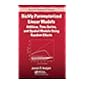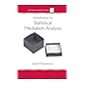Normal view MARC view ISBD view

# Richly parameterized linear models: additive, time series, and spatial models using random effects

Publisher: Boca Raton CRC Press 2014Description: xxxviii, 431 p.ISBN: 9781439866832.DDC classification: 519.536 Summary: A First Step toward a Unified Theory of Richly Parameterized Linear Models Using mixed linear models to analyze data often leads to results that are mysterious, inconvenient, or wrong. Further compounding the problem, statisticians lack a cohesive resource to acquire a systematic, theory-based understanding of models with random effects. Richly Parameterized Linear Models: Additive, Time Series, and Spatial Models Using Random Effects takes a first step in developing a full theory of richly parameterized models, which would allow statisticians to better understand their analysis results. The author examines what is known and unknown about mixed linear models and identifies research opportunities. The first two parts of the book cover an existing syntax for unifying models with random effects. The text explains how richly parameterized models can be expressed as mixed linear models and analyzed using conventional and Bayesian methods. In the last two parts, the author discusses oddities that can arise when analyzing data using these models. He presents ways to detect problems and, when possible, shows how to mitigate or avoid them. The book adapts ideas from linear model theory and then goes beyond that theory by examining the information in the data about the mixed linear model’s covariance matrices. Each chapter ends with two sets of exercises. Conventional problems encourage readers to practice with the algebraic methods and open questions motivate readers to research further. Supporting materials, including datasets for most of the examples analyzed, are available on the author’s website. (https://www.crcpress.com/Richly-Parameterized-Linear-Models-Additive-Time-Series-and-Spatial-Models/Hodges/p/book/9781439866832)
List(s) this item appears in: Laha
Tags from this library: No tags from this library for this title.
Item type Current location Item location Collection Call number Status Date due Barcode
Books Vikram Sarabhai Library
Slot 1427 (0 Floor, East Wing) Non-fiction 519.536 H6R4 (Browse shelf) Available 192274
##### Browsing Vikram Sarabhai Library Shelves , Collection code: Non-fiction Close shelf browser519.5​36 O5S7/2000 Spatial tessellations: concepts and applications of voronoi diagrams 519.536 H6M3 Methods and applications of linear models: regression and the analysis of variance 519.536 H6R4 Richly parameterized linear models: additive, time series, and spatial models using random effects 519.536 K3M8 Multiple regression and beyond: an introduction to multiple regression and structural equation modeling 519.536 K5M8 Multivariate nonparametric regression and visualization: with R and applications to finance 519.536 M2I6 Introduction to statistical mediation analysis (with CD)

1. Mixed Linear Models: Syntax, Theory, and Methods
An Opinionated Survey of Methods for Mixed Linear Models
Mixed linear models in the standard formulation
Conventional analysis of the mixed linear model
Bayesian analysis of the mixed linear model
Conventional and Bayesian approaches compared

2. Two More Tools: Alternative Formulation, Measures of Complexity
Alternative formulation: The "constraint-case" formulation
Measuring the complexity of a mixed linear model fit

3. Richly Parameterized Models as Mixed Linear Models
Penalized Splines as Mixed Linear Models
Penalized splines: Basis, knots, and penalty
More on basis, knots, and penalty
Mixed linear model representation

4. Additive Models and Models with Interactions
Additive models as mixed linear models
Models with interactions

5. Spatial Models as Mixed Linear Models
Geostatistical models
Models for areal data
Two-dimensional penalized splines

6. Time-Series Models as Mixed Linear Models
Example: Linear growth model
Dynamic linear models in some generality
Example of a multi-component DLM

7. Two Other Syntaxes for Richly Parameterized Models
Schematic comparison of the syntaxes
Gaussian Markov random fields
Likelihood inference for models with unobservables

8. From Linear Models to Richly Parameterized Models: Mean Structure
Preliminaries
Transforming variables
Case influence
Residuals

9. Puzzles from Analyzing Real Datasets
Four puzzles
Overview of the next three chapters

10. A Random Effect Competing with a Fixed Effect
Slovenia data: Spatial confounding
Kids and crowns: Informative cluster size

11. Differential Shrinkage
The simplified model and an overview of the results
Details of derivations
Conclusion: What might cause differential shrinkage?

12. Competition between Random Effects
Collinearity between random effects in three simpler models
Testing hypotheses on the optical-imaging data and DLM models
Discussion

13. Random Effects Old and New
Old-style random effects
New-style random effects
Practical consequences
Conclusion

14. Beyond Linear Models: Variance Structure
Mysterious, Inconvenient, or Wrong Results from Real Datasets
Periodontal data and the ICAR model
Periodontal data and the ICAR with two classes of neighbor pairs
Two very different smooths of the same data
Multiple maxima in posteriors and restricted likelihoods
Overview of the remaining chapters

15. Re-Expressing the Restricted Likelihood: Two-Variance Models
The re-expression
Examples
A tentative collection of tools

16. Exploring the Restricted Likelihood for Two-Variance Models
Which vj tell us about which variance?
Two mysteries explained

17. Extending the Re-Expressed Restricted Likelihood
Restricted likelihoods that can and can’t be re-expressed
Expedients for restricted likelihoods that can’t be re-expressed

18. Zero Variance Estimates
Some observations about zero variance estimates

19. Multiple Maxima in the Restricted Likelihood and Posterior
Restricted likelihoods with multiple local maxima
Posteriors with multiple modes

A First Step toward a Unified Theory of Richly Parameterized Linear Models

Using mixed linear models to analyze data often leads to results that are mysterious, inconvenient, or wrong. Further compounding the problem, statisticians lack a cohesive resource to acquire a systematic, theory-based understanding of models with random effects.

Richly Parameterized Linear Models: Additive, Time Series, and Spatial Models Using Random Effects takes a first step in developing a full theory of richly parameterized models, which would allow statisticians to better understand their analysis results. The author examines what is known and unknown about mixed linear models and identifies research opportunities.

The first two parts of the book cover an existing syntax for unifying models with random effects. The text explains how richly parameterized models can be expressed as mixed linear models and analyzed using conventional and Bayesian methods.

In the last two parts, the author discusses oddities that can arise when analyzing data using these models. He presents ways to detect problems and, when possible, shows how to mitigate or avoid them. The book adapts ideas from linear model theory and then goes beyond that theory by examining the information in the data about the mixed linear model’s covariance matrices.

Each chapter ends with two sets of exercises. Conventional problems encourage readers to practice with the algebraic methods and open questions motivate readers to research further. Supporting materials, including datasets for most of the examples analyzed, are available on the author’s website.